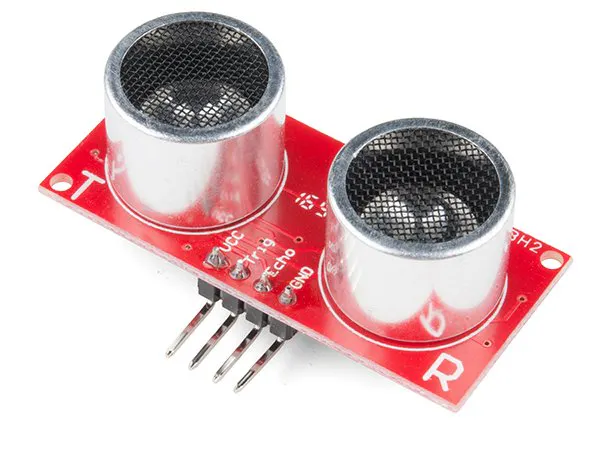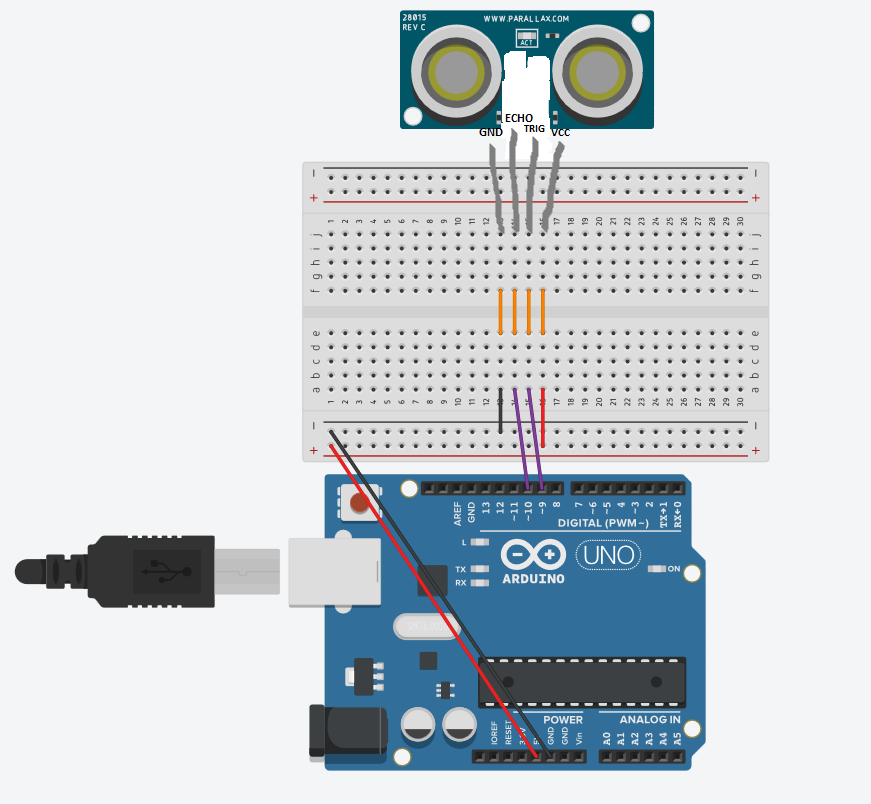# Getting Started with the HC-SR04 Ultrasonic sensor © CC BY-SA

A basic introduction to the low cost and accurate HC-SR04!

• 144,422 views
• 96 respects

## Apps and online services

In this project I will introduce you to the HC-SR04 Ultrasonic sensor. It works by sending sound waves from the transmitter, which then bounce off of an object and then return to the receiver. You can determine how far away something is by the time it takes for the sound waves to get back to the sensor. Let's get right to it!

### Connections

The connections are very simple:

• VCC to 5V
• GND to GND
• Trig to pin 9
• Echo to pin 10

You can actually connect Trig and Echo to whichever pins you want, 9 and 10 are just the ones I'm using.

### Code

First we define the pins that Trig and Echo are connected to.

``````const int trigPin = 9;
const int echoPin = 10;
``````

Then we declare 2 floats, duration and distance, which will hold the length of the sound wave and how far away the object is.

``````float duration, distance;
``````

Next, in the setup, we declare the Trig pin as an output, the Echo pin as an input, and start Serial communications.

``````void setup() {
pinMode(trigPin, OUTPUT);
pinMode(echoPin, INPUT);
Serial.begin(9600);
}
``````

Now, in the loop, what we do is first set the trigPin low for 2 microseconds just to make sure that the pin in low first. Then, we set it high for 10 microseconds, which sends out an 8 cycle sonic burst from the transmitter, which then bounces of an object and hits the receiver(Which is connected to the Echo Pin).

``````void loop() {
digitalWrite(trigPin, LOW);
delayMicroseconds(2);
digitalWrite(trigPin, HIGH);
delayMicroseconds(10);
digitalWrite(trigPin, LOW);
``````

When the sound waves hit the receiver, it turns the Echo pin high for however long the waves were traveling for. To get that, we can use a handy Arduino function called pulseIn(). It takes 2 arguments, the pin you are listening to(In our case, the Echo pin), and a state(HIGH or LOW). What the function does is waits for the pin to go whichever state you put in, starts timing, and then stops timing when it switches to the other state. In our case we would put HIGH since we want to start timing when the Echo pin goes high. We will store the time in the duration variable. (It returns the time in microseconds)

``````duration = pulseIn(echoPin, HIGH);
``````

Now that we have the time, we can use the equation speed = distance/time, but we will make it time x speed = distance because we have the speed. What speed do we have? The speed of sound, of course! The speed of sound is approximately 340 meters per second, but since the pulseIn() function returns the time in microseconds, we will need to have a speed in microseconds also, which is easy to get. A quick Google search for "speed of sound in centimeters per microsecond" will say that it is .0343 c/μS. You could do the math, but searching it is easier. Anyway, with that information, we can calculate the distance! Just multiply the duration by .0343 and then divide it by 2(Because the sound waves travel to the object AND back). We will store that in the distance variable.

``````distance = (duration*.0343)/2;
``````

The rest is just printing out the results to the Serial Monitor.

`````` Serial.print("Distance: ");
Serial.println(distance);
delay(100);
}
``````

Cover image by: Sparkfun

## Schematics• 27,967 views
• 41 respects

• 39,772 views
• 72 respects

#### How to Make an Ultrasonic Ruler with Arduino UNO and HC-SR04

Project showcase by Imperial_Proggramer

• 9,212 views
• 14 respects

#### Ultrasonic Sensor with Alarm, LCD and Temperature

Project tutorial by MichDragstar

• 29,517 views